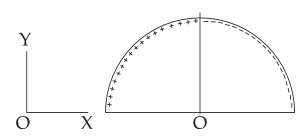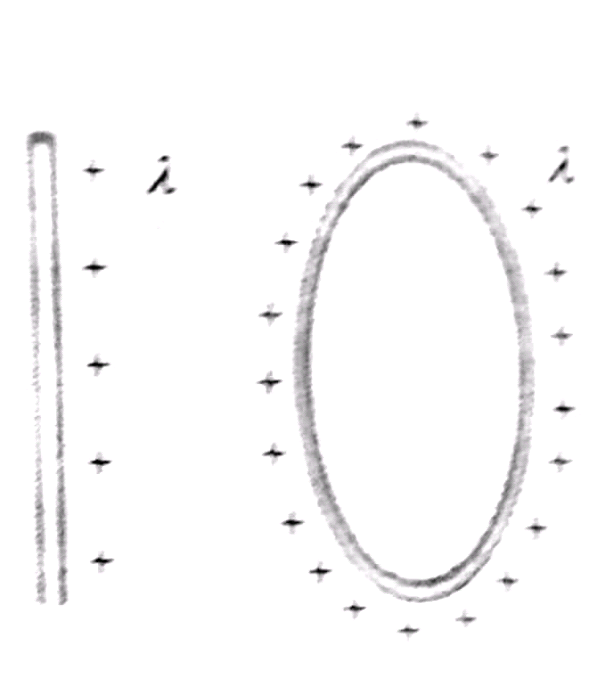#### A wire, of length L(=20 cm), is bent into a semi-circular arc. If the two equal halves, of the arc, were each to be uniformly charged with chargesCoulomb whereis the permittivity (in SI units) of free space] the net electric field at the centre O of the semi-circular arc would be :Option 1)Option 2)Option 3)Option 4)As we learnt in

Linear charge distribution -charge per unit length.- whereinwire, circulating ring ex.So due to each quarter section field intensitySo,Option 1)Incorrect Option

Option 2)Correct Option

Option 3)Incorrect Option

Option 4)Incorrect Option

#### Vakul